Author: Oscar Cronquist Article last updated on January 26, 2023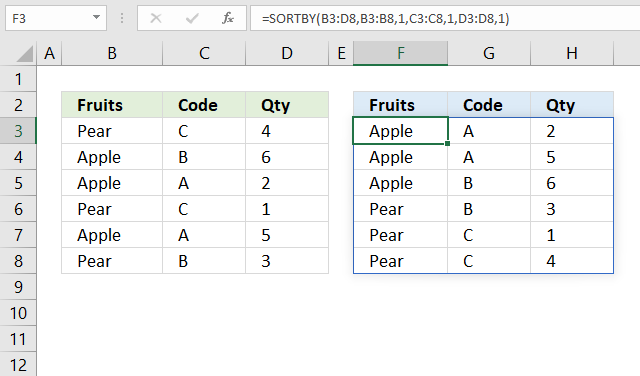The SORTBY function allows you to sort values from a cell range or array based on a corresponding cell range or array. It sorts values by column but keeps rows.

It is located in the Lookup and reference category and is only available to Excel 365 subscribers.

## 1. SORTBY Function Syntax

SORTBY(array, by_array1, [sort_order1], [by_array2, sort_order2],…)

## 2. SORTBY Function Arguments

 Argument Text array Required. Cell range or array. by_array1 Required. A cell range or array. [sort_order1] Optional. Sort order for argument by_array1. 1 - Ascending order (A to Z or small to large) -1 - descending order (Z to A or large to small). 1 is the default value if the argument is not specified. [by_array2] Optional. The cell range or array. [sort_order2] Optional. Sort order for argument [by_array2]. 1 - Ascending order (A to Z or small to large) -1 - descending order (Z to A or large to small). 1 is the default value if the argument is not specified.

## 3. SORTBY Function ExampleFormula in cell D3:

=SORTBY(B3:D8, B3:B8, 1, C3:C8, 1, D3:D8, 1)

The formula demonstrated in the image above sorts the data in cell range B3:D8 by column B and then column C and lastly column D in ascending order, and returns the sorted array to cell F3. This is not possible using the SORT function, the SORTBY function is more advanced.

## 4. What is a spilled array formula?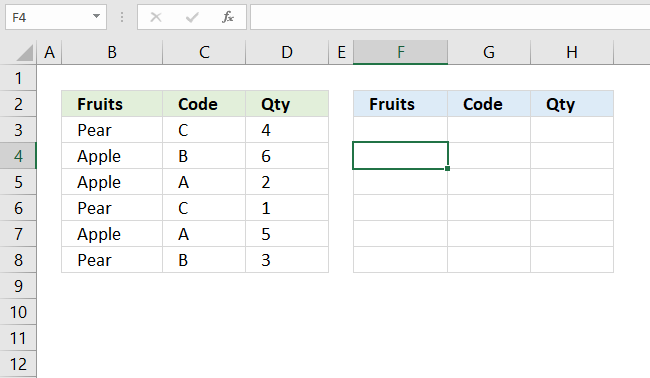Excel 365 automatically expands the output range based on the number of values in the array, this without requiring the user to enter the formula as an array formula.

This new behavior of Excel is called spilled array formula and is something only dynamic array formulas can do. Dynamic array formulas are only available to Excel 365 subscribers.

## 5. Why does the SORTBY function return a #SPILL! error?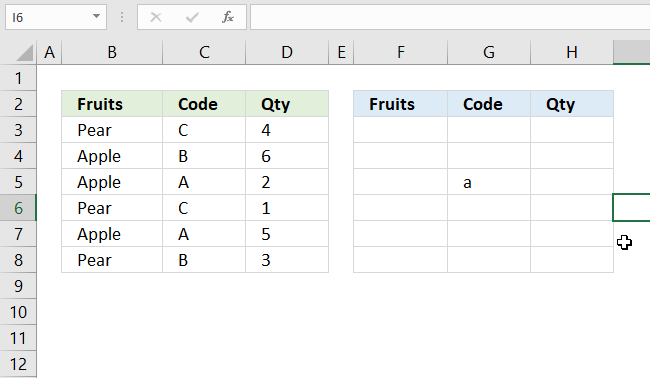If the needed cell range is populated by any other value a #SPILL! error is returned by the SORTBY function. You have two options:

• Remove value leaving the cell blank.
• Enter the dynamic formula in another cell that has empty adjacent cells.

## 6. Why does the SORTBY function return a #NAME! error?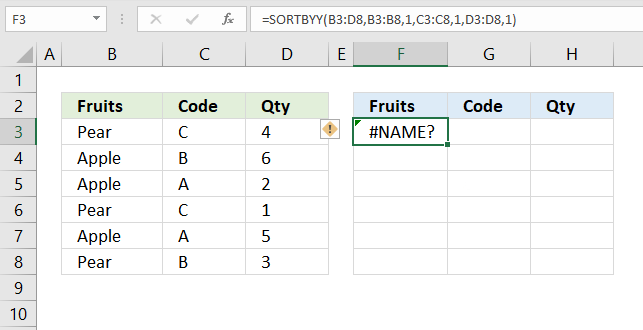If a cell returns #NAME! error you have either spelled the function name wrong or you use an incompatible Excel version.

The image above shows that I spelled the SORTBY function wrong in the formula bar, cell F3 displays #NAME! error.

Only Excel 365 subscription version supports the new dynamic array formula like the SORTBY function, older Excel versions like Excel 2019, 2016, 2013, 2010, 2007 and earlier versions do not support the SORTBY function.

Here is how to find out your Excel version: Get your Excel version

## 7. Does the SORTBY function differentiate between upper and lower letters?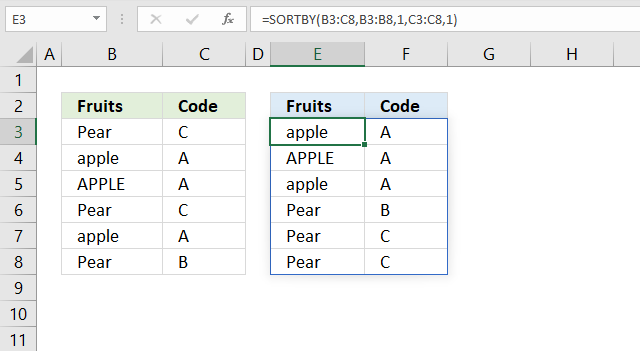No, the SORTBY function is not case sensitive. The image above shows that item "APPLE" in upper letters is not sorted differently than item "apple" in lower letters.

## 8. How to sort from A to Z?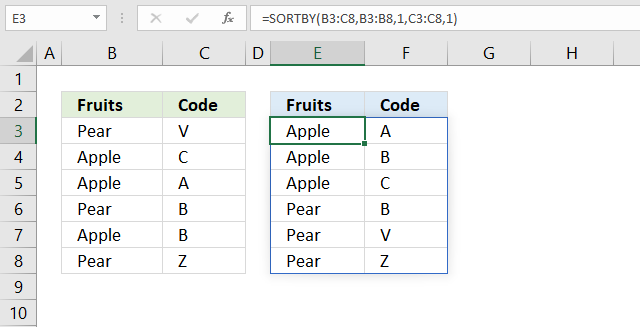=SORTBY(B3:C8,B3:B8,1,C3:C8,1)

SORTBY(array, by_array1, [sort_order1], [by_array2, sort_order2],…)

The third argument and then every other argument lets you choose the sort order. If omitted 1 is used.

1 -> Ascending order (A to Z or small to large)
-1 -> descending order (Z to A or large to small)

The formula above sorts values from cell range B3:C8 by cell range B3:B8 from A to Z, then by C3:C8 from A to Z.

## 9. How to sort from Z to A?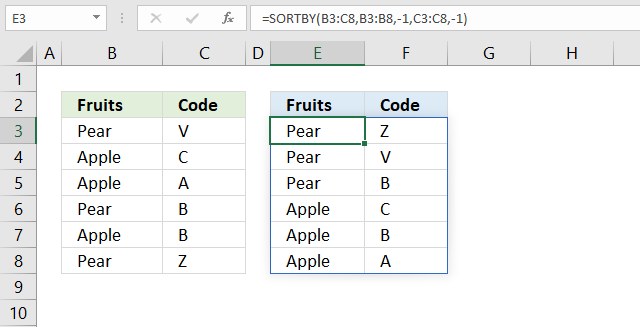=SORTBY(B3:C8,B3:B8,-1,C3:C8,-1)

SORTBY(array, by_array1, [sort_order1], [by_array2, sort_order2],…)

The third argument and then every other argument lets you choose the sort order. If omitted 1 is used.

1 -> Ascending order (A to Z or small to large)
-1 -> descending order (Z to A or large to small)

The formula above sorts values from cell range B3:C8 by cell range B3:B8 from Z to A, then by C3:C8 from Z to A.

## 10. How to sort from smallest to largest?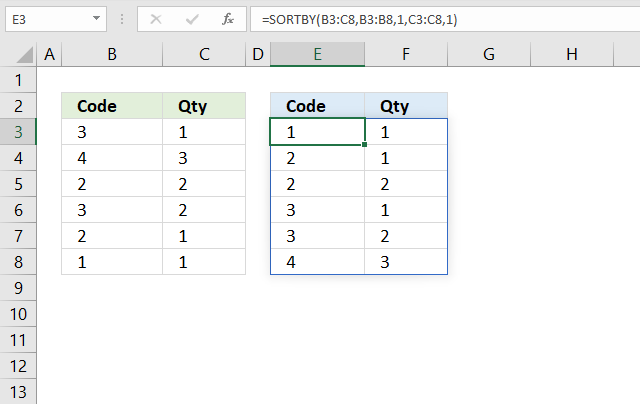=SORTBY(B3:C8,B3:B8,1,C3:C8,1)

SORTBY(array, by_array1, [sort_order1], [by_array2, sort_order2],…)

The third argument and then every other argument lets you choose the sort order. If omitted 1 is used.

1 -> Ascending order (A to Z or small to large)
-1 -> descending order (Z to A or large to small)

The formula above sorts values from cell range B3:C8 by cell range B3:B8 from small to large, then by C3:C8 from small to large.

## 11. How to sort from largest to smallest?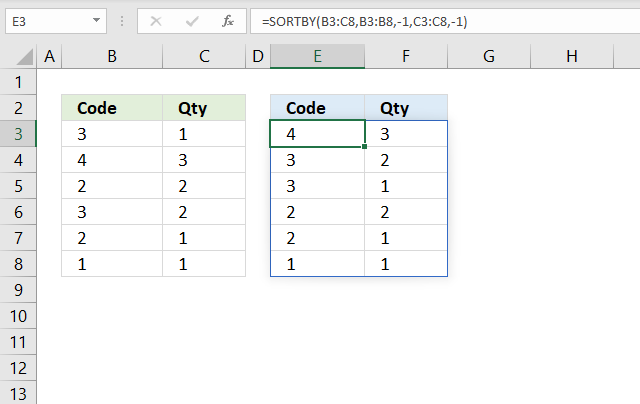=SORTBY(B3:C8,B3:B8,-1,C3:C8,-1)

SORTBY(array, by_array1, [sort_order1], [by_array2, sort_order2],…)

The third argument and then every other argument lets you choose the sort order. If omitted 1 is used.

1 -> Ascending order (A to Z or small to large)
-1 -> descending order (Z to A or large to small)

The formula above sorts values from cell range B3:C8 by cell range B3:B8 from large to small, then by C3:C8 from large to small.

## 12. How to sort by multiple columns?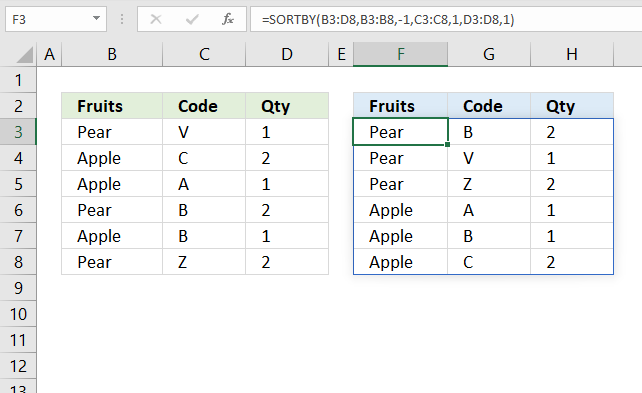The image above demonstrates a dynamic array formula in cell F3 that sorts values from cell range B3:D8 by column B in descending order, then by column C in ascending order, and lastly by column D in ascending order.

=SORTBY(B3:D8,B3:B8, -1, C3:C8, 1, D3:D8, 1)

SORTBY(array, by_array1, [sort_order1], [by_array2, sort_order2],…)

## 13. How to sort by another column?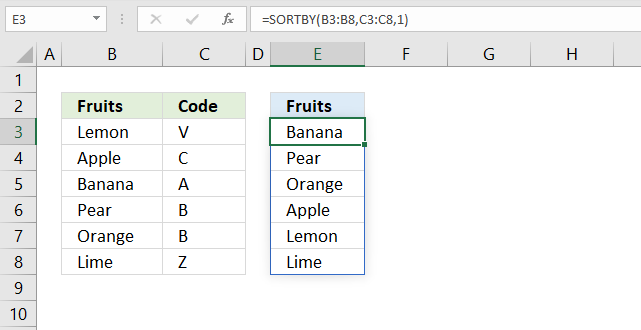The dynamic array formula in cell E3 sorts values from cell range B3:B8 by cell range C3:C8 in ascending order.

=SORTBY(B3:B8,C3:C8,1)

The SORTBY function allows you to sort values from a column by another column keeping rows intact. The image above demonstrates this, the fruits in column B are sorted by values in column C in ascending order (alphabetically).

SORTBY(array, by_array1, [sort_order1], [by_array2, sort_order2],…)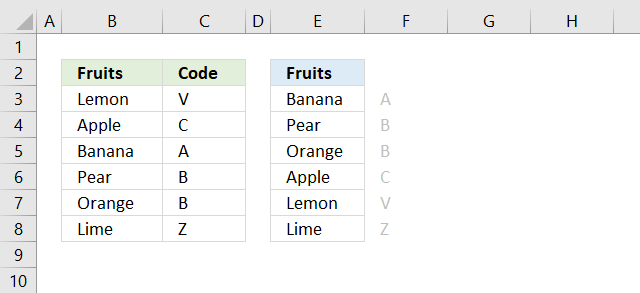## 14. How to sort by the first letter/digit? (Example 1)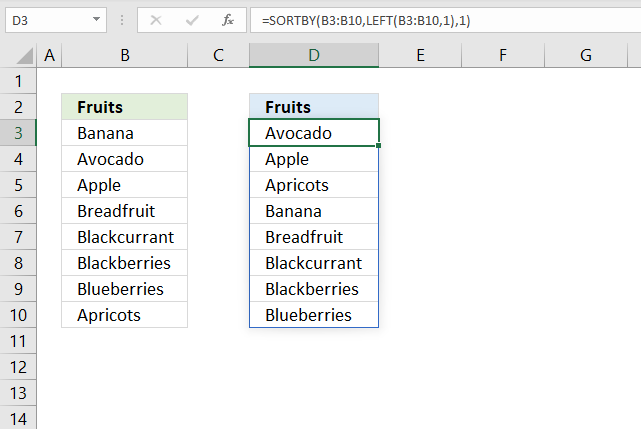Formula in cell D3:

=SORTBY(B3:B10,LEFT(B3:B10,1),1)

### Step 1 - Extract first character of each item

The LEFT function extracts a specific number of characters always starting from the left.

LEFT(text, [num_chars])

LEFT(B3:B10,1)

becomes

and returns

{"B"; "A"; "A"; "B"; "B"; "B"; "B"; "A"}

### Step 2 - Sort contents of cell range B3:B10 based on array

SORTBY(B3:B10,LEFT(B3:B10,1),1)

becomes

SORTBY(B3:B10,{"B"; "A"; "A"; "B"; "B"; "B"; "B"; "A"},1)

becomes

SORTBY({"Banana"; "Avocado"; "Apple"; "Breadfruit"; "Blackcurrant"; "Blackberries"; "Blueberries"; "Apricots"}, {"B"; "A"; "A"; "B"; "B"; "B"; "B"; "A"},1)

and returns

in cell D3 and cells below.## 14.1 How to sort by the first letter? (Example 2)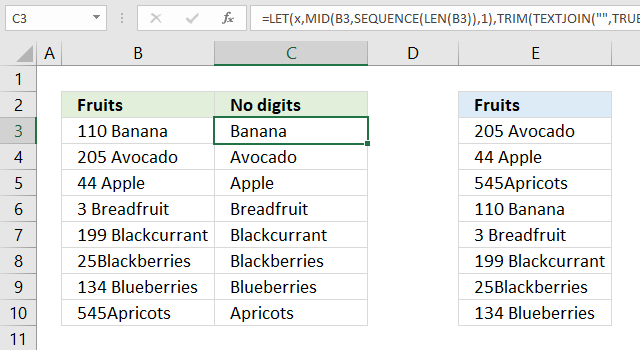The values in cell range B3:B10 don't begin with a letter, they begin with a number, we need another formula to extract the first found letter.

Formula in cell C3:

=LET(x, MID(B3, SEQUENCE(LEN(B3)), 1), TRIM(TEXTJOIN("", TRUE, IF(ISERROR((x*1)), x, ""))))

### Step 1 - Count characters

The LEN function counts the characters in a given value.

LEN(B3)

becomes

LEN("110 Banana")

and returns 10. "110 Banana" contains 10 characters excluding the double-quotes.

### Step 2 - Create a sequence from 1 to the number of characters in cell

The SEQUENCE function returns an array of numbers based on given limits.

SEQUENCE(rows,[columns],[start],[step])

SEQUENCE(LEN(B3))

becomes

SEQUENCE(10)

and returns {1; 2; 3; 4; 5; 6; 7; 8; 9; 10}. There are ten numbers in the array from 1 to 10.

### Step 3 - Extract each character in the cell

The MID function returns a part of a value based on the start character and number of characters.

MID(B3, SEQUENCE(LEN(B3)), 1)

becomes

MID(B3, {1; 2; 3; 4; 5; 6; 7; 8; 9; 10}, 1)

becomes

MID("110 Banana", {1; 2; 3; 4; 5; 6; 7; 8; 9; 10}, 1)

and returns the following array.

{"1"; "1"; "0"; " "; "B"; "a"; "n"; "a"; "n"; "a"}

### Step 4 - Multiply each value in the array with 1

The purpose of this step is to check if the character is a letter or a number. Multiplying a number works fine, however, multiplying a letter with a number results in an error.

MID(B3, SEQUENCE(LEN(B3)), 1)*1

becomes

{"1"; "1"; "0"; " "; "B"; "a"; "n"; "a"; "n"; "a"}*1

and returns

{1; 1; 0; #VALUE!; #VALUE!; #VALUE!; #VALUE!; #VALUE!; #VALUE!; #VALUE!}

### Step 5 - Check each value in array is an error

The ISERROR function returns true if the value is an error value and False if anything else.

ISERROR(MID(B3, SEQUENCE(LEN(B3)), 1)*1))

becomes

ISERROR({1; 1; 0; #VALUE!; #VALUE!; #VALUE!; #VALUE!; #VALUE!; #VALUE!; #VALUE!})

and returns

{FALSE; FALSE; FALSE; TRUE; TRUE; TRUE; TRUE; TRUE; TRUE; TRUE}

### Step 6 - Replace each error with corresponding character and replace numbers with nothing

The IF function replaces error values with the value itself and other values with nothing.

IF(ISERROR(MID(B3, SEQUENCE(LEN(B3)), 1)*1)), MID(B3, SEQUENCE(LEN(B3)), 1), "")

becomes

IF({FALSE; FALSE; FALSE; TRUE; TRUE; TRUE; TRUE; TRUE; TRUE; TRUE}, MID(B3, SEQUENCE(LEN(B3)), 1), "")

becomes

IF({FALSE; FALSE; FALSE; TRUE; TRUE; TRUE; TRUE; TRUE; TRUE; TRUE}, {"1"; "1"; "0"; " "; "B"; "a"; "n"; "a"; "n"; "a"}, "")

and returns

{""; ""; ""; " "; "B"; "a"; "n"; "a"; "n"; "a"}.

### Step 7 - Join all remaining values in array

The TEXTJOIN function concatenates all values in an array.

TEXTJOIN("", TRUE, IF(ISERROR((MID(B3, SEQUENCE(LEN(B3)), 1)*1)), MID(B3, SEQUENCE(LEN(B3)), 1), ""))

becomes

TEXTJOIN("", TRUE, {""; ""; ""; " "; "B"; "a"; "n"; "a"; "n"; "a"})

and returns "Banana".

### Step 8 - Remove leading and trailing blanks

The TRIM function removes leading and trailing blanks.

TRIM(TEXTJOIN("", TRUE, IF(ISERROR((MID(B3, SEQUENCE(LEN(B3)), 1)*1)), MID(B3, SEQUENCE(LEN(B3)), 1), "")))

becomes

TRIM("Banana")

and returns "Banana".

### Step 9 - Shorten formula

The LET function lets you shorten big formulas.

TRIM(TEXTJOIN("", TRUE, IF(ISERROR((MID(B3, SEQUENCE(LEN(B3)), 1)*1)), MID(B3, SEQUENCE(LEN(B3)), 1), "")))

becomes

LET(x, MID(B3, SEQUENCE(LEN(B3)), 1), TRIM(TEXTJOIN("", TRUE, IF(ISERROR((x*1)), x, ""))))Formula in cell E3:

=SORTBY(B3:B10, LEFT(C3:C10, 1), 1)

## 14.2 How to sort by the first digit? (Example 3)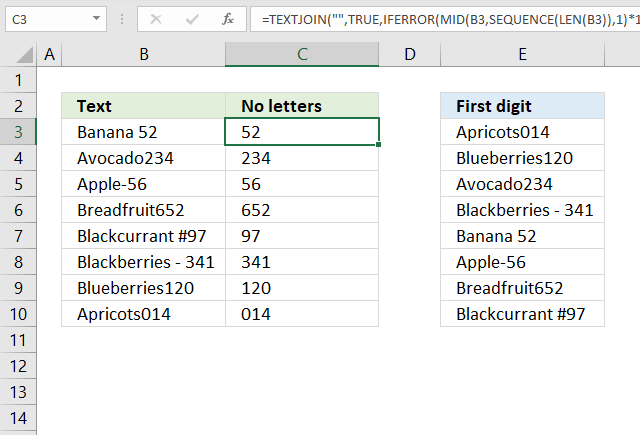Formula in cell C3:

=TEXTJOIN("",TRUE,IFERROR(MID(B3, SEQUENCE(LEN(B3)),1)*1,""))

### Step 1 - Count characters in cell

The LEN function counts the characters in a given value.

LEN(B3)

becomes

LEN("Banana 52")

and returns 9. There are nine characters in "Banana 52" excluding the double-quotes.

### Step 2 - Create an array with numbers

The SEQUENCE function returns an array of numbers based on given limits.

SEQUENCE(rows,[columns],[start],[step])

SEQUENCE(LEN(B3))

becomes

SEQUENCE(9)

and returns {1; 2; 3; 4; 5; 6; 7; 8; 9}

### Step 3 - Extract each character

The MID function returns a substring from a string based on the starting position and the number of characters you want to extract.

Here is the syntax: MID(textstart_numnum_chars)

MID(B3, SEQUENCE(LEN(B3)),1)

becomes

MID(B3, {1; 2; 3; 4; 5; 6; 7; 8; 9},1)

becomes

MID("Banana 52", {1; 2; 3; 4; 5; 6; 7; 8; 9},1)

and returns {"B";"a";"n";"a";"n";"a";" ";"5";"2"}.

### Step 4 - Identify numbers

This step multiplies all values in the array with 1, a letter returns an error value and a number returns a number.

MID(B3, SEQUENCE(LEN(B3)),1)*1

becomes

{"B";"a";"n";"a";"n";"a";" ";"5";"2"}*1

and returns {#VALUE!; #VALUE!; #VALUE!; #VALUE!; #VALUE!; #VALUE!; #VALUE!; 5; 2}.

### Step 5 - Create boolean values True or False

The IFERROR function returns a specified value if a value is an error and nothing for everything else.

IFERROR(MID(B3, SEQUENCE(LEN(B3)),1)*1,"")

becomes

IFERROR({#VALUE!; #VALUE!; #VALUE!; #VALUE!; #VALUE!; #VALUE!; #VALUE!; 5; 2},"")

and returns {"";"";"";"";"";"";"";5;2}.

### Step 6 - Join values in array

The TEXTJOIN function combines text strings, here is the syntax: TEXTJOIN(delimiterignore_emptytext1[text2], ...)

TEXTJOIN("",TRUE,IFERROR(MID(B3, SEQUENCE(LEN(B3)),1)*1,""))

becomes

TEXTJOIN("",TRUE,{"";"";"";"";"";"";"";5;2})

and returns "52" in cell C3.## 15. How to sort by absolute number?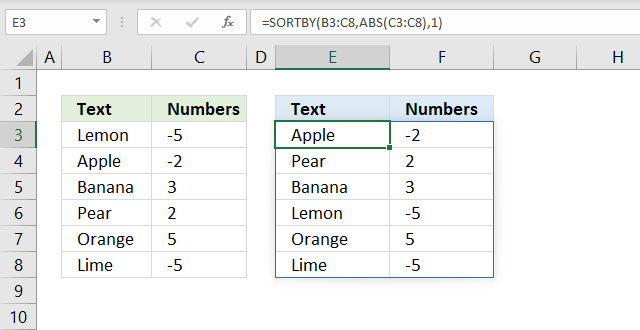Formula in cell E3:

=SORTBY(B3:C8,ABS(C3:C8),1)

### Step 1 - Remove negative signs

The ABS function converts negative numbers to positive numbers.

ABS(C3:C8)

becomes

ABS({-5;-2;3;2;5;-5})

and returns {5; 2; 3; 2; 5; 5}.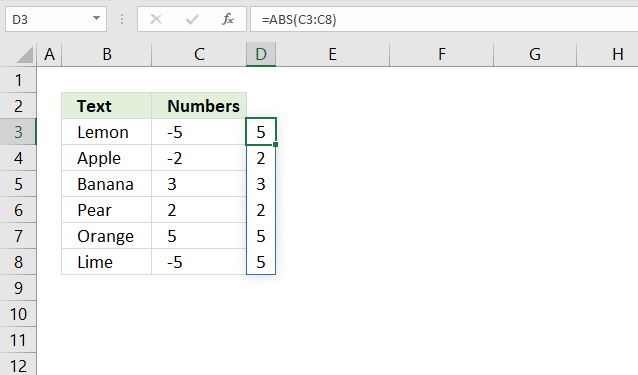### Step 2 - Sort by numbers from small to large

SORTBY(B3:C8, ABS(C3:C8), 1)

becomes

SORTBY(B3:C8, {5; 2; 3; 2; 5; 5}, 1)

becomes

SORTBY({"Lemon",-5; "Apple",-2; "Banana",3; "Pear",2; "Orange",5; "Lime",-5}, {5; 2; 3; 2; 5; 5}, 1)

and returns

{"Apple",-2; "Pear",2; "Banana",3; "Lemon",-5; "Orange",5; "Lime",-5} in cell E3.## 16. How to sort by the last word in a cell?The image above demonstrates a dynamic array formula in cell D3 that sorts values from cell range B3:B8 by the last word in each cell.

Formula in cell D3:

=SORTBY(B3:B8, TRIM(RIGHT(SUBSTITUTE(B3:B8, " ", REPT(" ", 200)), 200)))

### Step 1 - Repeat blank

The REPT function repeats a text string based on a given number.

REPT(" ", 200)

returns a text string with 200 space characters.

### Step 2 - Substitute blank with repeated blanks

The SUBSTITUTE function replaces all instances of a specific text string in a value.

SUBSTITUTE(B3:B8, " ", REPT(" ", 200))

becomes

SUBSTITUTE({"Lemon pie"; "Apple sauce"; "Banana split"; "Pear cream dessert"; "Orange jello"; "Lime cream"}," ",REPT(" ",200))

and returns

{"Lemon pie";"Apple sauce";"Banana split";"Pear cream dessert";"Orange jello";"Lime cream"}.

WordPress removes duplicate space characters, I hope you get the idea anyway.

### Step 3 - Extracts 100 characters starting from the right

The RIGHT function extracts a specific number of characters always starting from the right.

RIGHT(SUBSTITUTE(B3:B8, " ", REPT(" ", 200)), 200)

returns

{" pie";" sauce";" split";" dessert";" jello";" cream"}

All blanks are not shown, WordPress removes duplicate space characters.

### Step 4 - Remove remaining blanks

TRIM(RIGHT(SUBSTITUTE(B3:B8, " ", REPT(" ", 200)), 200))

returns

{"pie";"sauce";"split";"dessert";"jello";"cream"}

### Step 5 - Sort B3:B8 by last word

SORTBY(B3:B8, TRIM(RIGHT(SUBSTITUTE(B3:B8, " ", REPT(" ", 200)), 200)))

becomes

SORTBY(B3:B8, {"pie";"sauce";"split";"dessert";"jello";"cream"})

becomes

SORTBY({"Lemon pie"; "Apple sauce"; "Banana split"; "Pear cream dessert"; "Orange jello"; "Lime cream"}, {"pie";"sauce";"split";"dessert";"jello";"cream"})

and returns

{"Lime cream"; "Pear cream dessert"; "Orange jello"; "Lemon pie"; "Apple sauce"; "Banana split"}## 17. How to sort by first name?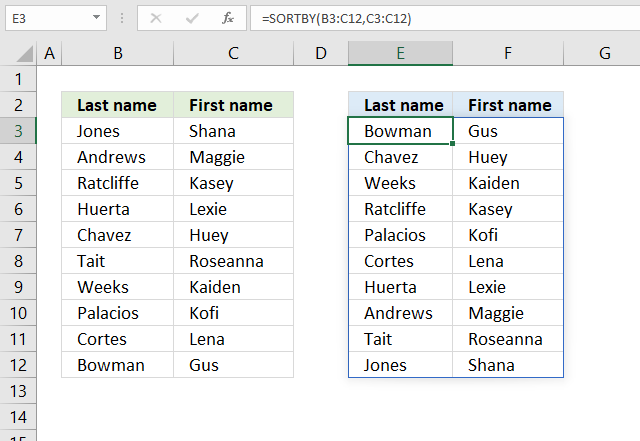The image above demonstrates a dynamic array formula in cell E3 that sorts values from cell range B3:B8 by the first name.

Formula in cell E3:

=SORTBY(B3:C12, C3:C12)

## 18. How to sort by last name?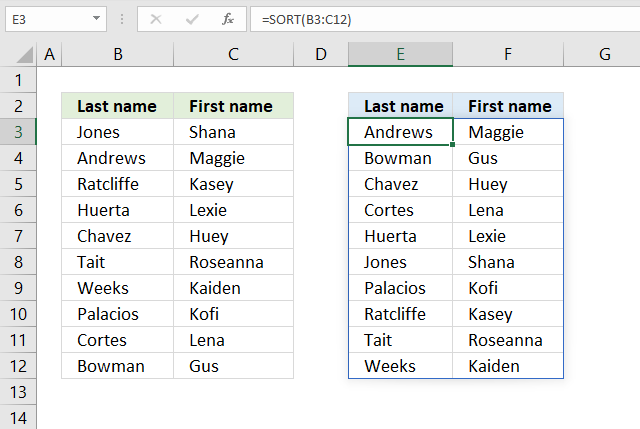The easiest way to sort last names and first names in the image above is to use the SORT function.

Formula in cell D3:

=SORT(B3:C12)

It sorts the array by the first column from A to Z if you omit all optional arguments.

SORT(array, [sort_index], [sort_order], [by_col])

## 19. How to sort by a custom list?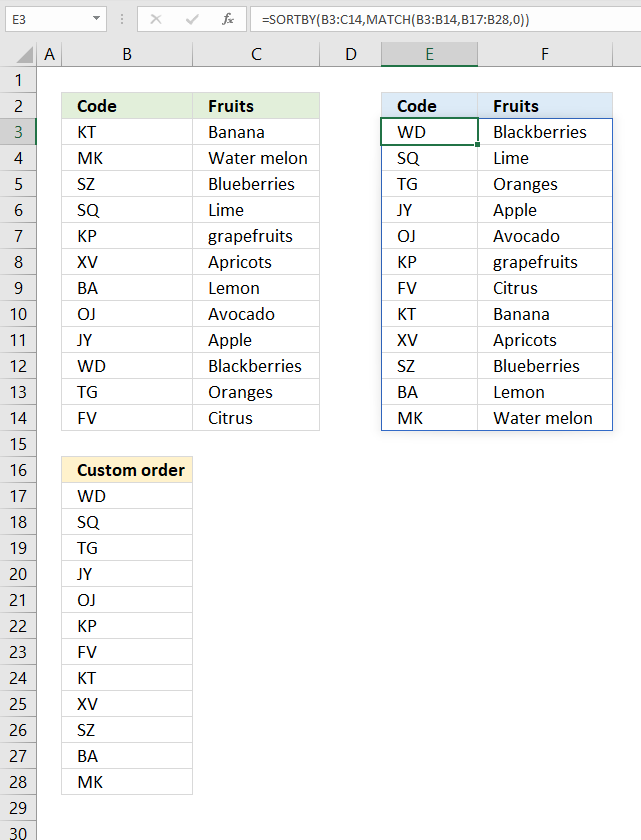The formula in cell E3 rearranges the rows in cell range B3:C14 based on a custom list specified in cell range B17:B28.

Formula in cell E3:

=SORTBY(B3:C14, MATCH(B3:B14, B17:B28, 0))

### Step 1 - Find position of item in a custom list

The MATCH function returns the relative position of an item in an array or cell reference that matches a specified value in a specific order.

MATCH(lookup_value, lookup_array, [match_type])

MATCH(B3:B14, B17:B28, 0)

becomes

MATCH({"KT"; "MK"; "SZ"; "SQ"; "KP"; "XV"; "BA"; "OJ"; "JY"; "WD"; "TG"; "FV"},{"WD"; "SQ"; "TG"; "JY"; "OJ"; "KP"; "FV"; "KT"; "XV"; "SZ"; "BA"; "MK"},0)

and returns {8; 12; 10; 2; 6; 9; 11; 5; 4; 1; 3; 7}. These numbers represent the position in the custom list and they tell you how to sort the values.

### Step 2 - Sort cell range B3:C14 based on numbers

SORTBY(B3:C14,MATCH(B3:B14,B17:B28,0))

becomes

SORTBY(B3:C14, {8; 12; 10; 2; 6; 9; 11; 5; 4; 1; 3; 7})

becomes

SORTBY({"KT","Banana"; "MK","Water melon"; "SZ","Blueberries"; "SQ","Lime"; "KP","grapefruits"; "XV","Apricots"; "BA","Lemon"; "OJ","Avocado"; "JY","Apple"; "WD","Blackberries"; "TG","Oranges"; "FV","Citrus"}, {8; 12; 10; 2; 6; 9; 11; 5; 4; 1; 3; 7})

and returns

{"WD","Blackberries"; "SQ","Lime"; "TG","Oranges"; "JY","Apple"; "OJ","Avocado"; "KP","grapefruits"; "FV","Citrus"; "KT","Banana"; "XV","Apricots"; "SZ","Blueberries"; "BA","Lemon"; "MK","Water melon"}

in cell E3 and cells below.## 20. How to sort by month?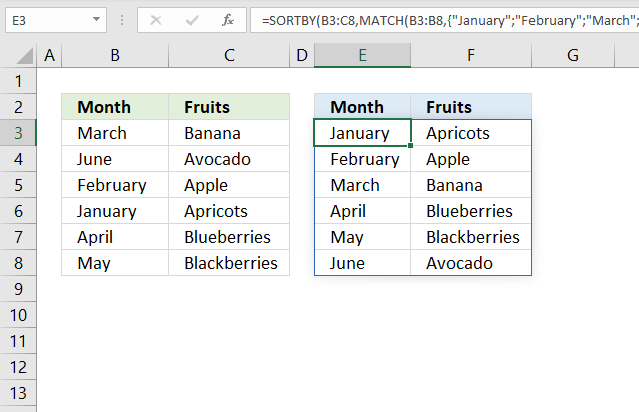Formula in cell E3:

=SORTBY(B3:C8, MATCH(B3:B8, {"January"; "February"; "March"; "April"; "May"; "June"; "July"; "August"; "September"; "October"; "November"; "December"}, 0))

### Step 1 - Convert values to numbers

The MATCH function allows you to calculate a position in a given array or cell range based on a lookup value. It returns a number that represents the position in the array.

MATCH(B3:B8, {"January"; "February"; "March"; "April"; "May"; "June"; "July"; "August"; "September"; "October"; "November"; "December"}, 0)

becomes

MATCH({"March"; "June"; "February"; "January"; "April"; "May"}, {"January"; "February"; "March"; "April"; "May"; "June"; "July"; "August"; "September"; "October"; "November"; "December"}, 0)

and returns

{3; 6; 2; 1; 4; 5}

The first number in the array is 3, it corresponds to March in cell range B3:B8. March is the third month. The second month in cell range B3:B8 is June, the corresponding number is 6. June is the sixth month.

### Step 2 - Sort array based on numbers

SORTBY(B3:C8, MATCH(B3:B8, {"January"; "February"; "March"; "April"; "May"; "June"; "July"; "August"; "September"; "October"; "November"; "December"}, 0))

becomes

SORTBY(B3:C8, {3; 6; 2; 1; 4; 5})

becomes

SORTBY({"March", "Banana"; "June", "Avocado"; "February", "Apple"; "January", "Apricots"; "April", "Blueberries"; "May", "Blackberries"}, {3; 6; 2; 1; 4; 5})

and returns

{"January", "Apricots"; "February", "Apple"; "March", "Banana"; "April", "Blueberries"; "May", "Blackberries"; "June", "Avocado"}## 21. Sort by word length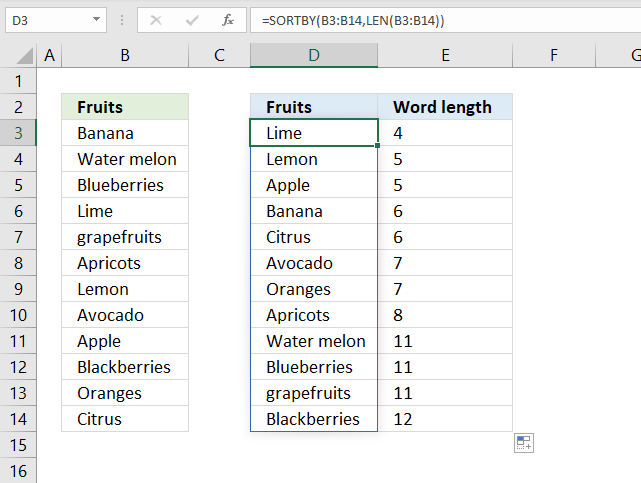The image above demonstrates a dynamic array formula in cell D3 that sorts the cell values in cell range B3:B14 by word length.

Formula in cell D3:

=SORTBY(B3:B14, LEN(B3:B14))

### Step 1 - Calculate word length

The LEN function counts the number of characters in a cell or text string.

LEN(B3:B14)

becomes

LEN({"Banana"; "Water melon"; "Blueberries"; "Lime"; "grapefruits"; "Apricots"; "Lemon"; "Avocado"; "Apple"; "Blackberries"; "Oranges"; "Citrus"})

and returns

{6; 11; 11; 4; 11; 8; 5; 7; 5; 12; 7; 6}

### Step 2 - Sort cell range B3:B14 by word length number

SORTBY(B3:B14, LEN(B3:B14))

becomes

SORTBY(B3:B14, {6; 11; 11; 4; 11; 8; 5; 7; 5; 12; 7; 6})

becomes

SORTBY({"Banana"; "Water melon"; "Blueberries"; "Lime"; "grapefruits"; "Apricots"; "Lemon"; "Avocado"; "Apple"; "Blackberries"; "Oranges"; "Citrus"}, {6; 11; 11; 4; 11; 8; 5; 7; 5; 12; 7; 6})

and returns

{"Lime"; "Lemon"; "Apple"; "Banana"; "Citrus"; "Avocado"; "Oranges"; "Apricots"; "Water melon"; "Blueberries"; "grapefruits"; "Blackberries"}

in cell range D3:D14.## 22. Sort in random order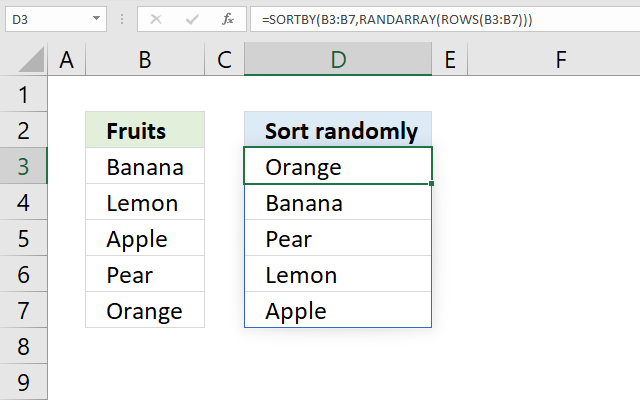Formula in cell D3:

=SORTBY(B3:B7, RANDARRAY(ROWS(B3:B7)))

### Step 1 - Count the number of rows in cell range B3:B7

The ROWS function returns the number of rows from a cell range or array.

ROWS(B3:B7)

becomes

ROWS({"Banana";"Lemon";"Apple";"Pear";"Orange"})

and returns 5. There are five rows in cell range B3:B7.

### Step 2 - Create random values between 0 (zero) and 1

The RANDARRAY function returns an array of randomized numbers, you can specify the size of the array, and if decimals or whole numbers are to be returned, also the min and max numbers.

RANDARRAY([rows],[columns],[min],[max],[whole_number])

We are going to specify only the row argument which makes the RANDARRAY function return random numbers based on the ROWS function.

RANDARRAY(ROWS(B3:B7))

becomes

RANDARRAY(5)

and returns 5 random decimal numbers.

{0.853939211740133; 0.581193997533527; 0.1410253631491; 0.747189425872485; 0.394921711301841}

### Step 3 - Sort values in B3:B7 based on random values

SORTBY(B3:B7,RANDARRAY(ROWS(B3:B7)))

becomes

SORTBY(B3:B7, {0.853939211740133; 0.581193997533527; 0.1410253631491; 0.747189425872485; 0.394921711301841})

becomes

SORTBY({"Banana"; "Lemon"; "Apple"; "Pear"; "Orange"}, {0.853939211740133; 0.581193997533527; 0.1410253631491; 0.747189425872485; 0.394921711301841})

and returns values from cell range B3:B7 randomly.

{"Lemon";"Banana";"Orange";"Apple";"Pear"}

My formula is inspired by a formula made by David Hager found here: Excel #8 – Making a Bingo Card Using Only Formulas with Dynamic Array Functions

## 23. How to sort by week number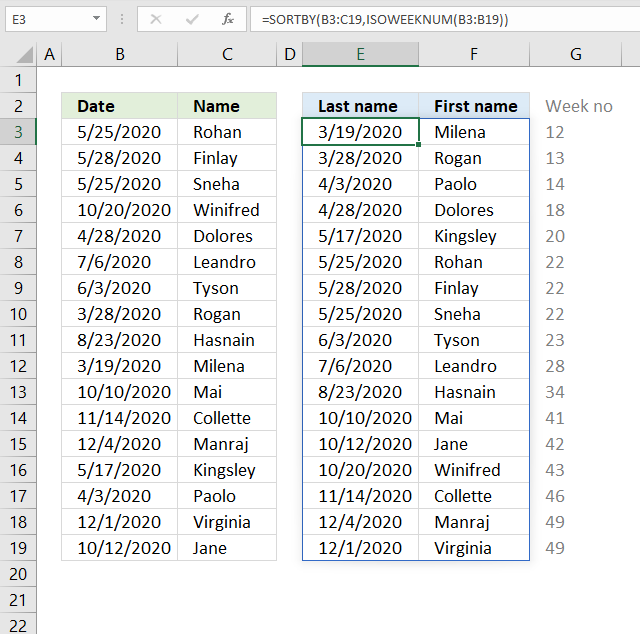The image above shows a dynamic array formula that sorts values from cell range B3:C19 by the corresponding week number.

Formula in cell E3:

=SORTBY(B3:C19, ISOWEEKNUM(B3:B19))

### Step 1 - Calculate week number

The ISOWEEKNUM function calculates a number of the ISO week number of the year for a given date.

ISOWEEKNUM(B3:B19)

returns

{22; 22; 22; 43; 18; 28; 23; 13; 34; 12; 41; 46; 49; 20; 14; 49; 42}

### Step 2 - Sort by week number

SORTBY(B3:C19, ISOWEEKNUM(B3:B19))

becomes

SORTBY(B3:C19, {22; 22; 22; 43; 18; 28; 23; 13; 34; 12; 41; 46; 49; 20; 14; 49; 42})

and returns

{43909,"Milena"; 43918,"Rogan"; 43924,"Paolo"; 43949,"Dolores"; 43968,"Kingsley"; 43976,"Rohan"; 43979,"Finlay"; 43976,"Sneha"; 43985,"Tyson"; 44018,"Leandro"; 44066,"Hasnain"; 44114,"Mai"; 44116,"Jane"; 44124,"Winifred"; 44149,"Collette"; 44169,"Manraj"; 44166,"Virginia"}.## 24. How to sort by quarter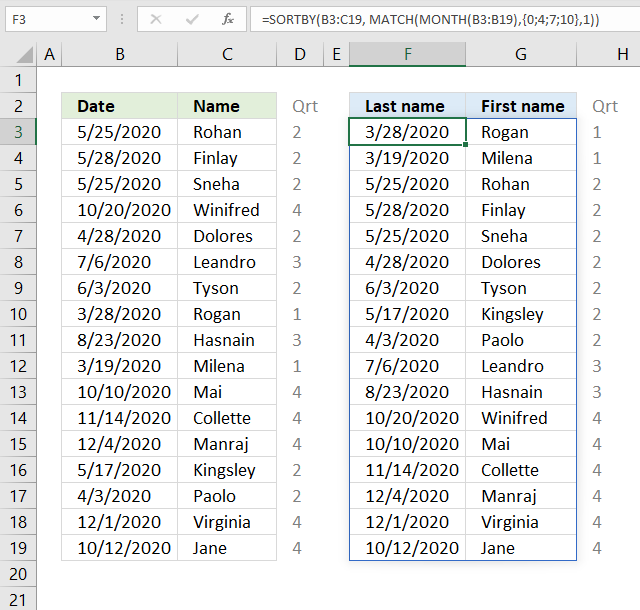The image above shows a dynamic array formula that calculates quarters based on dates in column B, it then sorts values from cell range B3:V19 by the quarters from small to large.

Formula in cell E3:

=SORTBY(B3:C19, MATCH(MONTH(B3:B19), {0;4;7;10}, 1))

### Step 1 - Calculate month number

The MONTH function returns a number from 1 to 12 from an Excel date representing the position. 1 - January, ... , 12 - December.

MONTH(B3:B19)

becomes

MONTH({43976; 43979; 43976; 44124; 43949; 44018; 43985; 43918; 44066; 43909; 44114; 44149; 44169; 43968; 43924; 44166; 44116})

and returns

{5; 5; 5; 10; 4; 7; 6; 3; 8; 3; 10; 11; 12; 5; 4; 12; 10}

### Step 2 - Calculate quarter

The MATCH function returns a number representing the quarter, in this specific example.

MATCH(MONTH(B3:B19), {0;4;7;10}, 1)

becomes

MATCH({5; 5; 5; 10; 4; 7; 6; 3; 8; 3; 10; 11; 12; 5; 4; 12; 10}, {0;4;7;10}, 1)

and returns

{2; 2; 2; 4; 2; 3; 2; 1; 3; 1; 4; 4; 4; 2; 2; 4; 4}.

January -> 1 -> Quarter 1
February -> 2 -> Quarter 1
March -> 3 -> Quarter 1
April -> 4 -> Quarter 2
May -> 5 -> Quarter 2
June -> 6 -> Quarter 2
July -> 7 -> Quarter 3
August -> 8 -> Quarter 3
September -> 9 -> Quarter 3
October -> 10 -> Quarter 4
November -> 11 -> Quarter 4
December -> 12 -> Quarter 4

### Step 3 - Sort by quarter

SORTBY(B3:C19, MATCH(MONTH(B3:B19), {0;4;7;10}, 1))

becomes

SORTBY(B3:C19, {2; 2; 2; 4; 2; 3; 2; 1; 3; 1; 4; 4; 4; 2; 2; 4; 4})

and returns

{43918,"Rogan"; 43909,"Milena"; 43976,"Rohan"; 43979,"Finlay"; 43976,"Sneha"; 43949,"Dolores"; 43985,"Tyson"; 43968,"Kingsley"; 43924,"Paolo"; 44018,"Leandro"; 44066,"Hasnain"; 44124,"Winifred"; 44114,"Mai"; 44149,"Collette"; 44169,"Manraj"; 44166,"Virginia"; 44116,"Jane"}

in cell F3.## 25. How to sort based on row count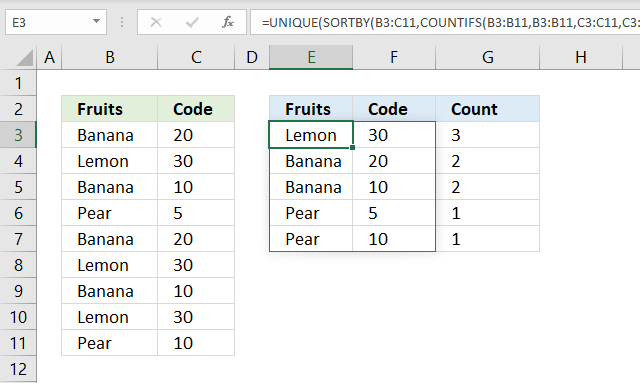The image above demonstrates a formula in cell E3 that extracts unique distinct rows from cell range B3:C11 sorted by row count.

For example, row 3 contains "Banana" and "20". Row 7 contains "Banana" and "20" as well, which makes the total row count 2 for that particular row.

The dynamic array formula in cell G3 returns 2 in cell G4 which corresponds to "Banana" and "20" in cell range E4:F4.

Formula in cell E3:

=UNIQUE(SORTBY(B3:C11, COUNTIFS(B3:B11, B3:B11, C3:C11, C3:C11), -1))

### Step 1 - Count duplicate rows

The COUNTIFS function calculates the number of cells across multiple ranges that equals all given conditions.

COUNTIFS(criteria_range1, criteria1, [criteria_range2, criteria2]…)

We have two columns and must use two pairs of arguments to be able to count rows. The result is an array containing numbers for each corresponding row.

COUNTIFS(B3:B11, B3:B11, C3:C11, C3:C11)

becomes

COUNTIFS({"Banana"; "Lemon"; "Banana"; "Pear"; "Banana"; "Lemon"; "Banana"; "Lemon"; "Pear"}, {"Banana"; "Lemon"; "Banana"; "Pear"; "Banana"; "Lemon"; "Banana"; "Lemon"; "Pear"}, {20; 30; 10; 5; 20; 30; 10; 30; 10}, {20; 30; 10; 5; 20; 30; 10; 30; 10})

and returns {2; 3; 2; 1; 2; 3; 2; 3; 1}.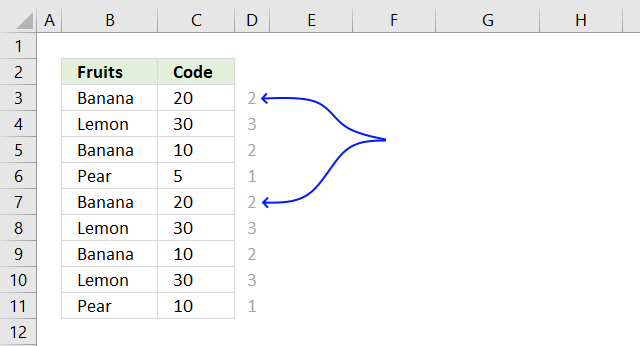The first number in the array corresponds to the first row and so on. 2 means that there is a duplicate row in cell range B3:C11, the blue arrows show where that duplicate row is located.

### Step 2 - Sort array based on row count

SORTBY(array, by_array1, [sort_order1], [by_array2, sort_order2],…)

The SORTBY function sorts values from cell range B3:C11 by the result array from the COUNTIFS function from large to small.

SORTBY(B3:C11, COUNTIFS(B3:B11, B3:B11, C3:C11, C3:C11), -1)

becomes

SORTBY(B3:C11, {2; 3; 2; 1; 2; 3; 2; 3; 1}, -1)

becomes

SORTBY({"Banana",20; "Lemon",30; "Banana",10; "Pear",5; "Banana",20; "Lemon",30; "Banana",10; "Lemon",30; "Pear",10}, {2; 3; 2; 1; 2; 3; 2; 3; 1}, -1)

and returns

{"Lemon",30; "Lemon",30; "Lemon",30; "Banana",20; "Banana",10; "Banana",20; "Banana",10; "Pear",5; "Pear",10}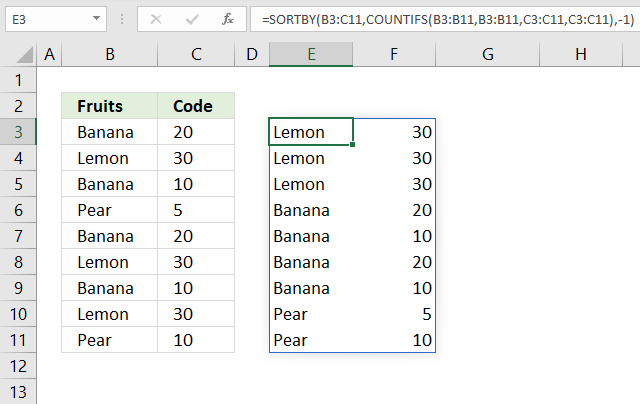### Step 3 - Extract distinct rows

The UNIQUE function extracts unique distinct rows from the array.

UNIQUE(array,[by_col],[exactly_once])

UNIQUE(SORTBY(B3:C11, COUNTIFS(B3:B11, B3:B11, C3:C11, C3:C11), -1))

becomes

UNIQUE({"Lemon",30; "Lemon",30; "Lemon",30; "Banana",20; "Banana",10; "Banana",20; "Banana",10; "Pear",5; "Pear",10})

and returns

{"Lemon",30,3; "Banana",20,2; "Banana",10,2; "Pear",5,1; "Pear",10,1}

in cell E3.Formula in cell G3:

=COUNTIFS(\$B\$3:\$B\$11, E3:E7, \$C\$3:\$C\$11, F3:F7)

I recommend a Pivot Table if you have a very large data set and experience a slow responding worksheet, it is lightning fast.